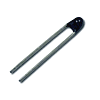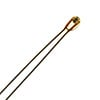📦Free shipping over \$10 in most countries and regions!

NTC Thermistor

What are NTC Thermistors?NTC stands for "Negative Temperature Coefficient". NTC thermistors are resistors with a negative temperature coefficient, which means that the resistance decreases with increasing temperature. They are primarily used as resistive temperature sensors and current-limiting devices. The temperature sensitivity coefficient is about five times greater than that of silicon temperature sensors (silistors) and about ten times greater than that of resistance temperature detectors (RTDs). NTC sensors are typically used in a range from −55 to +200 °C.

The non-linearity of the relationship between resistance and temperature exhibited by NTC resistors posed a great challenge when using analog circuits to accurately measure temperature. However, rapid development of digital circuits solved that problem through enabling computation of precise values by interpolating lookup tables or by solving equations which approximate a typical NTC curve.

NTC Thermistor Definition
An NTC thermistor is a thermally sensitive resistor for which the resistance exhibits a large, precise and predictable decrease as the core temperature of the resistor increases over the operating temperature range.

Characteristics of NTC Thermistors

Unlike RTDs (Resistance Temperature Detectors), which are made from metals, NTC thermistors are generally made of ceramics or polymers. Different materials used in the manufacture of NTC thermistors result in different temperature responses, as well as other different performance characteristics.

Temperature response

Most NTC thermistors are typically suitable for use within a temperature range between −55 and 200 °C, where they give their most precise readings. There are special families of NTC thermistors that can be used at temperatures approaching absolute zero (-273.15 °C) as well as those specifically designed for use above 150 °C.

The temperature sensitivity of an NTC sensor is expressed as “percentage change per degree C" or "percentage change per degree K". Depending on the materials used and the specifics of the production process, the typical values of temperature sensitivities range from -3% to -6% / °C.Characteristic NTC curve

As can be seen from the figure, the NTC thermistors have a much steeper resistance-temperature slope compared to platinum alloy RTDs, which translates to better temperature sensitivity. Even so, RTDs remain the most accurate sensors with their accuracy being ±0.5 % of the measured temperature, and they are useful in the temperature range between -200 and 800 °C, a much wider range than that of NTC temperature sensors.

Comparison to other temperature sensors

Compared to RTDs, the NTC thermistors have a smaller size, faster response, greater resistance to shock and vibration at a lower cost. They are slightly less precise than RTDs. The precision of NTC thermistors is similar to thermocouples. However thermocouples, can withstand very high temperatures (in the order of 600 °C) and are used in these applications instead of NTC thermistors. Even so, NTC thermistors provide greater sensitivity, stability and accuracy than thermocouples at lower temperatures and are used with less additional circuitry and therefore at a lower total cost. The cost is additionally lowered by the lack of need for signal conditioning circuits (amplifiers, level translators, etc.) that are often needed when dealing with RTDs and always needed for thermocouples.

Self-heating effect

The self-heating effect is a phenomenon that takes place whenever there is a current flowing through the NTC thermistor. Since the thermistor is basically a resistor, it dissipates power as heat when there is a current flowing through it. This heat is generated in the thermistor core and affects the precision of the measurements. The extent to which this happens depends on the amount of current flowing, the environment (whether it is a liquid or a gas, whether there is any flow over the NTC sensor and so on), the temperature coefficient of the thermistor, the thermistor’s total area and so on. The fact that the resistance of the NTC sensor and therefore the current through it depends on the environment is often used in liquid presence detectors such as those found in storage tanks.

Heat capacity

The heat capacity represents the amount of heat required to increase the temperature of the thermistor by 1 °C and is usually expressed in mJ/°C. Knowing the precise heat capacity is of great importance when using an NTC thermistor sensor as an inrush-current limiting device, as it defines the response speed of the NTC temp sensor.

Curve Selection and Calculation

The thermistor selection process must take care of the thermistor’s Dissipation Constant, Thermal Time Constant, Resistance value, Resistance-Temperature curve and Tolerances, to mention the most important factors.

Since the relationship between resistance and temperature (the R-T curve) is highly nonlinear, certain approximations have to be utilized in practical system designs.

First-order approximation

One approximation, and the simplest to use, is the first-order approximation which states that:

$$\Delta R = k · \Delta T$$

Where k is the negative temperature coefficient, ΔT is the temperature difference, and ΔR is the resistance change resulting from the change in temperature. This first-order approximation is only valid for a very narrow temperature range, and can only be used for such temperatures where k is nearly constant throughout the whole temperature range.

Beta formula

Another equation gives satisfying results, being accurate to ±1 °C over the range of 0 to +100°C. It is dependent on a single material constant β which can be obtained by measurements. The equation can be written as:

$$R(T) = R(T_0) · e^{\beta (\frac{1}{T} - \frac{1}{T_0})}$$

Where R(T) is the resistance at the temperature T in Kelvin, R(T0) is a reference point at temperature T0. The Beta formula requires a two-point calibration, and it is typically not more accurate than ±5 °C over the complete useful range of the NTC thermistor.

Steinhart-Hart equation

The best approximation known to date is the Steinhart-Hart formula, published in 1968:

$$\frac{1}{T} = A + B · ln(R) + C · (ln(R))^3$$

Where ln R is the natural logarithm of the resistance at temperature T in Kelvin, and A, B and C are coefficients derived from experimental measurements. These coefficients are usually published by thermistor vendors as part of the datasheet. The Steinhart-Hart formula is typically accurate to around ±0.15 °C over the range of -50 to +150 °C, which is plenty for most applications. If superior accuracy is required, the temperature range must be reduced and accuracy of better than ±0.01 °C over the range of 0 to +100 °C is achievable.

Choosing the right approximation

The choice of the formula used to derive the temperature from the resistance measurement needs to be based on available computing power, as well as actual tolerance requirements. In some applications, a first-order approximation is more than enough, while in others not even the Steinhart-Hart equation fulfills the requirements, and the thermistor has to be calibrated point by point, making a large number of measurements and creating a lookup table.

Construction and Properties of NTC Thermistors

Materials typically involved in the fabrication of NTC resistors are platinum, nickel, cobalt, iron and oxides of silicon, used as pure elements or as ceramics and polymers. NTC thermistors can be classified into three groups, depending on the production process used.These NTC thermistors are made from platinum alloy lead wires directly sintered into the ceramic body. They generally offer fast response times, better stability and allow operation at higher temperatures than Disk and Chip NTC sensors, however they are more fragile. It is common to seal them in glass, to protect them from mechanical damage during assembly and to improve their measurement stability. The typical sizes range from 0.075 – 5 mm in diameter.

Disk and Chip thermistorsThese NTC thermistors have metalized surface contacts. They are larger and, as a result, have slower reaction times than bead type NTC resistors. However, because of their size, they have a higher dissipation constant (power required to raise their temperature by 1 °C). Since the power dissipated by the thermistor is proportional to the square of the current, they can handle higher currents much better than bead type thermistors. Disk type thermistors are made by pressing a blend of oxide powders into a round die and then sintering at high temperatures. Chips are usually fabricated by a tape-casting process where a slurry of material is spread out as a thick film, dried and cut into shape. The typical sizes range from 0.25 to 25 mm in diameter.

Glass encapsulated NTC thermistorsThese are NTC temperature sensors sealed in an airtight glass bubble. They are designed for use with temperatures above 150 °C, or for printed circuit board mounting, where ruggedness is a must. Encapsulating a thermistor in glass improves the stability of the sensor and protects the sensor from the environment. They are made by hermetically sealing bead type NTC resistors into a glass container. The typical sizes range from 0.4 to 10 mm in diameter.

Typical Applications

NTC thermistors are used in a broad spectrum of applications. They are used to measure temperature, control temperature, and compensate for temperature. They can also be used to detect the absence or presence of a liquid, as current limiting devices in power supply circuits, for temperature monitoring in automotive applications, and in many more applications. NTC sensors can be divided into three groups, depending on the electrical characteristic exploited in an application.

Resistance-temperature characteristic

Applications based on the resistance-temperature characteristic include temperature measurement, control, and compensation. These also include situations in which an NTC thermistor is used so that the temperature of the NTC temp sensor is related to some other physical phenomena. This group of applications requires that the thermistor operates in a zero-power condition, meaning that the current through it is kept as low as possible, to avoid heating the probe.

Current-time characteristic

Applications based on current-time characteristic are: time delay, inrush-current limiting, surge suppression, and many more. These characteristics are related to the heat capacity and dissipation constant of the NTC thermistor used. The circuit usually relies on the NTC thermistor heating up due to the current passing through it. At one point it will trigger some sort of change in the circuit, depending on the application in which it is used.

Voltage-current characteristic

Applications based on the voltage-current characteristic of a thermistor generally involve changes in the environmental conditions or circuit variations which result in changes in the operating point on a given curve in the circuit. Depending on the application, this can be used for current limiting, temperature compensation or temperature measurements.

NTC Thermistor Symbol

The following symbol is used for a negative temperature coefficient thermistor, according to the IEC standard.NTC thermistor (IEC standard)

If you need NTC Thermistors:
👉 100K Ohm NTC Thermistors(5Pcs)
👉 100K Ohm NTC Thermistors(10Pcs)
👉 NTC 3950 100K Thermistor(5Pcs)
👉 NTC Thermistor Sensor Probe(5Pcs)

More Electronic Component，welcome to HALJIA!